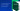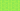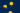Skip to contentPricingLoginSign up# Straight Line Depreciation: Which method of depreciation should you be using?

Because different companies consider different factors when calculating depreciation (the decline of value in a fixed asset over time), there are a range of different depreciation methods that you may decide to use in your company accounts. We’ve put together a brief introduction to each one, so you can choose the best depreciation method for your business’s needs.

## Straight line method of depreciation

The straight-line method of depreciation, also referred to as the fixed instalment method, is probably the most widely used method of calculating depreciation. In the straight-line method of depreciation, the value of the asset depreciates by an equal amount in each accounting period, up to the end of its useful life when the asset is reduced to zero or its remaining salvage value. Want to know how to calculate depreciation rate using the straight-line method? Simple – you can use the following formula:

Straight-Line Depreciation = (Purchase Price – Salvage Value) / Useful Life

## Reducing balance method of depreciation

Also known as the diminishing balance method, the reducing balance method of depreciation is referred to as an “accelerated” depreciation method because it expenses a greater proportion of the asset’s value early in its useful life, before recording incrementally smaller depreciation expenses in later years. This makes it a great choice for technology assets, such as computing equipment or mobile phones. The reducing balance depreciation method formula is as follows:

Reducing Balance Depreciation = (Value at Beginning of the Year x Depreciation Rate) / 100

## Sum of digits method for depreciation

Like the reducing balance method of depreciation, the sum of digits method for depreciation (often referred to as the sum of years’ digits method) allocates more depreciation in the earlier years of an asset’s useful life, which makes it an accelerated depreciation method. You can use the formula below to calculate the sum of digits method for depreciation:

Sum of Digits Depreciation = Depreciable Cost x (Remaining Useful Life / Sum of Years’ Digits)

Note – Calculate the sum of years’ digits with the following, additional formula:

Sum of Years’ Digits = (Useful Life x (Useful Life + 1)) / 2

## Units of production method of depreciation

While the other depreciation methods we’ve mentioned in this article base their assessment of the decline in an asset’s value on time, the units of production method centres it on the amount of work or activity that the asset experiences. Essentially, each unit of production has an equal expense rate, meaning that depreciation is tied directly to the output capacity of the asset. When there’s higher usage, there’s a higher depreciation rate, while periods of low usage lead to lower depreciation rates. As such, it’s best suited to manufacturing or processing equipment. If you want to make use of the units of production depreciation method, you can try the following formula:

Units of Production Depreciation = (Number of Units Produced / Life in Number of Units) x (Cost – Salvage Value)

## Double declining balance method of depreciation

Finally, there’s the double declining balance depreciation method. Another accelerated method, the double declining balance method leads to a much greater proportion of the asset’s value being expensed in the early years of its usable life. This makes it an ideal choice for assets that are much more productive when you first buy them, such as a computer or laptop. Want to know how to calculate the double declining balance method? Use this formula (but note, you’ll need to calculate the straight-line depreciation rate first):

Double Declining Depreciation = 2 x Straight-Line Depreciation Rate x Value at Beginning of the Year

## How to choose which depreciation method to use

It’s always difficult to know which depreciation method to apply when you’re doing your accounts, and there are many advantages and disadvantages associated with each method. That’s why it’s a good idea to focus your attention on the nature of your business’s assets. Do they become less useful at an even rate? If so, it’s best to use the straight-line method of depreciation. What happens if they make a sudden drop in value? In that case, you may be best served with the double declining balance method. Remember, depreciation can have a significant effect on cash flow, so it helps to get this decision right from the start.

## We can help

GoCardless helps you automate payment collection, cutting down on the amount of admin your team needs to deal with when chasing invoices. Find out how GoCardless can help you with ad hoc payments or recurring payments.

GoCardless is used by over 55,000 businesses around the world. Learn more about how you can improve payment processing at your business today.

Learn moreSign Up

Interested in automating the way you get paid? GoCardless can help

Contact salesContact Us

Sales

Contact sales

Support

Seen 'GoCardless Ltd' on your bank statement? Learn more

GoCardless Ltd., Sutton Yard, 65 Goswell Road, London, EC1V 7EN, United Kingdom

GoCardless (company registration number 07495895) is authorised by the Financial Conduct Authority under the Payment Services Regulations 2017, registration number 597190, for the provision of payment services. GoCardless SAS (23-25 Avenue Mac-Mahon, Paris, 75017, France), an affiliate of GoCardless Ltd (company registration number 834 422 180, R.C.S. PARIS), is authorised by the ACPR (French Prudential Supervision and Resolution Authority), Bank Code (CIB) 17118, for the provision of payment services.